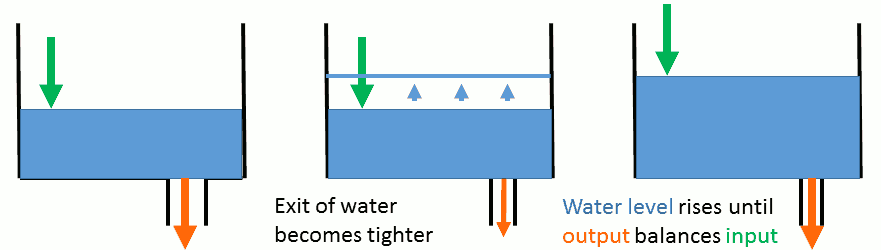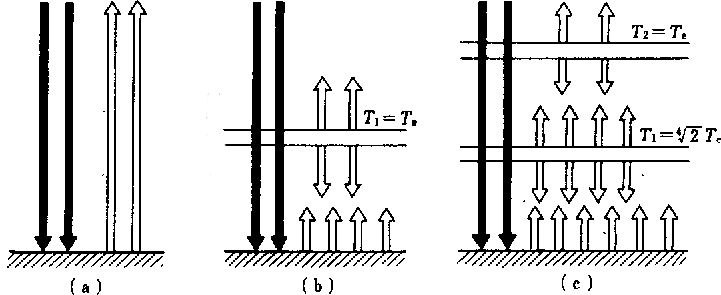﻿ Zero-dimensional energy balance model of the climate system

Zero-dimensional energy balance model of the climate system

Physics-based models of the climate system

(There are many types of models of the climate system, whose complexity is various.)

Zero-dimensional energy balance model

• The simplest physics-based model of the climate system.
• Governing equation: (only) conservation of energy.
• Spatial dimension: spatial variation in east-west, north-south, up-down dimensions are all ignored. (Any quantity is assumed to be uniform in east-west and north-south directions, and to have a constant profile except a single-value offset in the vertical direction.)
• Energy stock is assumed to be (constant) heat capacity multiplied by temperature.
• Energy input is absorption of solar radiation (mainly visible and near infra-red).
• Energy output is emission of terrestrial radiation (thermal infra-red).
• For convenience, quantities are expressed per unit area of the earth's surface.
• C dT/dt = (1/4) (1 - αp) S - Fout
• C: (effective) heat capacity of the climate system per unit area [J/(m2 K)]
• T: representative temperature of the climate system [K]
• 1/4: ratio between the cross-sectional area and the surface area of a sphere
• αp: reflectance of solar radiation by the earth as a whole, or "planetary albedo". [non-dimensional]
• S: solar irradiance (energy flow per unit time per unit area) at a plane perpendicular to the sun-earth line. So-called "solar constant". [W/m2]
• Fout: radiance (energy flow per unit time per unit area) of terrestrial radiation emitted to space [W/m2]
• T does not change in time. (S and αp are assumed to be constant as well.)
• 0 = (1/4) (1 - αp) S - Fout• Steady state, Assumption 1: Fout is blackbody radiation at temperature T.
• 0 = (1/4) (1 - αp) S - σB T4
• Assume that S = 1.36 × 103 W/m2 [Corrected after lecture], and αp = 0.30 (according to average values of modern observations).
• Then, T = 254 K = approx. -19 ℃
• This T is the representative temperature of the terrestrial radiation. (Note: Terrestrial radiation in the real world is a mixture of radiation emitted by the land and sea surface, by cloud top, and by atmospheric gases. It is not uniform in space.) This T is called "effective radiative temperature of the earth" Te.
• Steady state, Assumption 2: Fout is {blackbody radiation at temperature T} multiplied by εp.
• 0 = (1/4) (1 - αp) S - εp σB T4
• εp may be (temporarily) called "apparent planetary emissivity".
• εp T4 = Te4
• εp(1/4) T = Te
• T = Te / εp(1/4) = εp(-1/4) Te
• If εp < 1, T > Te.
• Smaller εp ... "stronger greenhouse effect"
• If T = 287 K (14 ℃, actual global average surface air temperture), how much is εp ?

A bath-tub model

• A stock-and-flow system as well as a feedback system
• Useful for explaining gradual change between quasi-steady states
• Governing equation: conservation of mass of water
• Stock: mass of water, proportional to water level
• Inflow: mass flow of water, independent of water level
• Outflow: mass flow of water, increasing function of water level
• For a given constant inflow and a given outflow function, a steady state exists. From any non-steady state, the system will approach the steady state.
• What will happen if the inflow changes suddenly, and then kept at the new constant value?
• What will happen if the outflow function changes suddenly, and then kept at the new constant value?Analogy of the climate system with the bath-tub model (preliminary)

• Stock: energy stock, proportional to temperature
• Inflow: absorbed part of incoming solar radiation
• Outflow: outgoing terrestrial radiation
• "Water exit tightened" ... εp decreased ... "greenhouse effect enhanced".

Causes of global climate change that can be represented by 0-dimensional energy balance models

• Note: There may be causes of climate change that cannot be represented by 0-dim. models.
• Change of inflow of energy
• Change of energy flow emitted from the sun (Change of S)
• Change of matter in the space between the sun and the earth that interacts with radiation (unknown, but perhaps not important)
• Change of geometric configuration between the sun and the earth (important in 10000-year time scale)
• Change of absorption and reflection of solar radiation by the climate system (Change of αp)
• Change of outflow of energy
• Change of "apparent planetary emissivity" εp, in other words, change of "greenhouse effect"

What causes change in reflectivity of solar radiation? How they depend on temperarture?

• Surface of land and ocean
• Snow and ice (feedback [to temperature])
• Vegetation, land cover (external forcing [as a 1st approx.])
• Suspended liquid and solid particles in the atmosphere
• Clouds [H2O] (feedback)
• Aerosols (external forcing)

What causes change in "apparent planetary emissivity" of terrestrial radiation? ...

• Gaseous components of the atmosphere ("greenhouse gases")
• Water vapor [H2O] (feedback)
• Carbon dioxide [CO2] etc. (external forcing)
• Suspended liquid and solid particles in the atmosphere (same as above)

A "plate glass" model of the "greenhouse effect" of the atmosphere

• (Note that this is not the dominant effect of the actual greenhouse made of plate glass.)
• Zero-dimensional energy balance model, steady state.
• Sum of 2 black arrows corresponds to (1/4) (1 - αp) S .
• The ground is assumed to be blackbody.
• (a): the same as "assumption 1" above. Ground temperature T = Te.
• The atmosphere is assumed to be concentrated in a plate whose vertical dimension is negligibly small. Also, it is assumed to be transparent for solar radiation and opaque (same as blackbody) for terrestrial radiation.
• (b): one plate
• Temperature of the atmospheric plate = Te
• Ground temperature / Te = 2(1/4) = sqrt(sqrt(2)) = approx. 1.2
• (c): 2 plates ... Ground temperature = 3(1/4) Te.
• More plates, higher ground temperature.
• The surface temperature of Venus (ca. 740 K) can be explained this way.
• This model can produce indefinitely high temperature, but that is not real, because the separation of wavelength between solar and terrestrial radiation will not hold at very high ground temperature.2019-May-16
MASUDA Kooiti